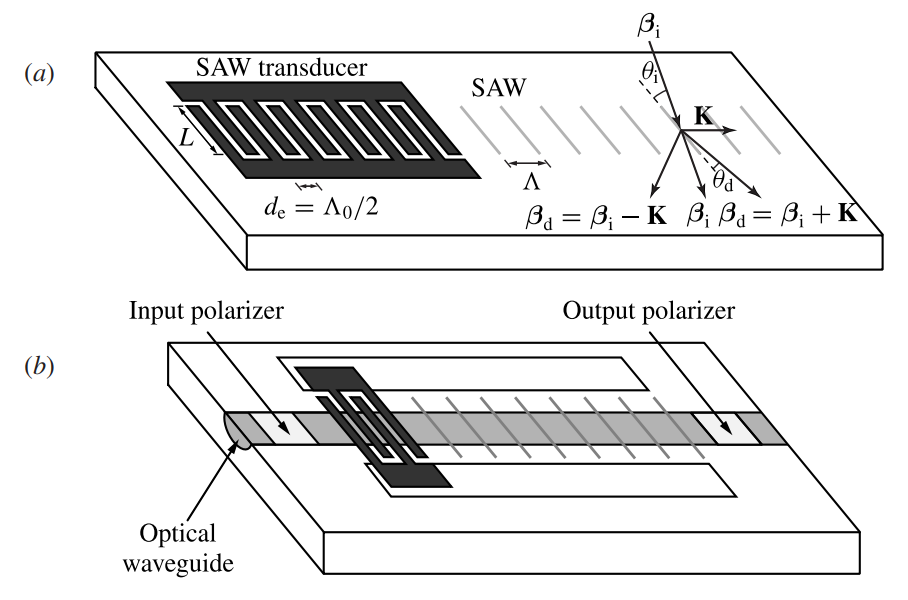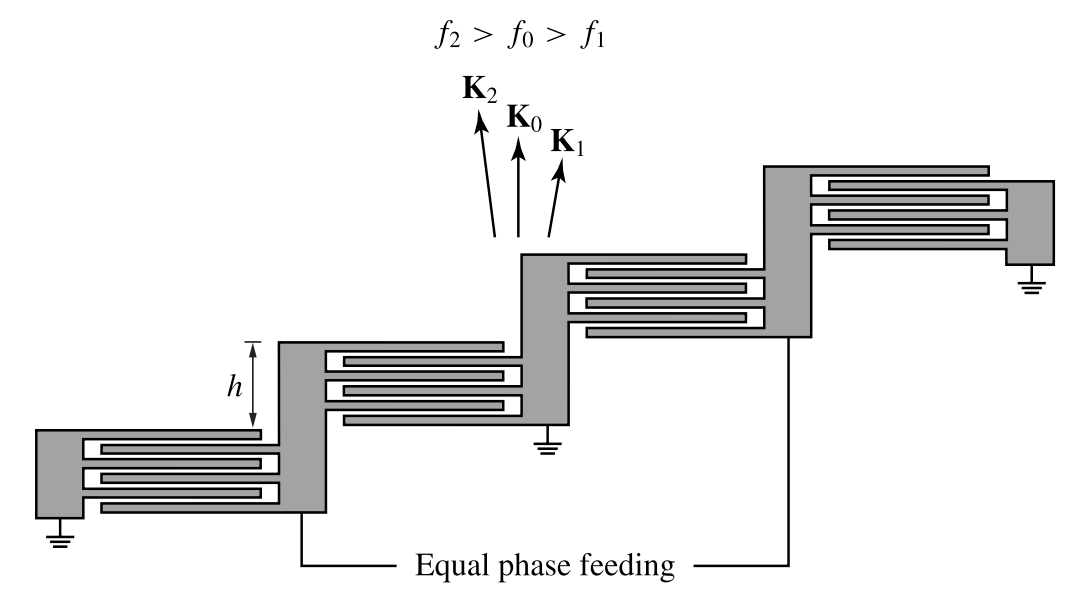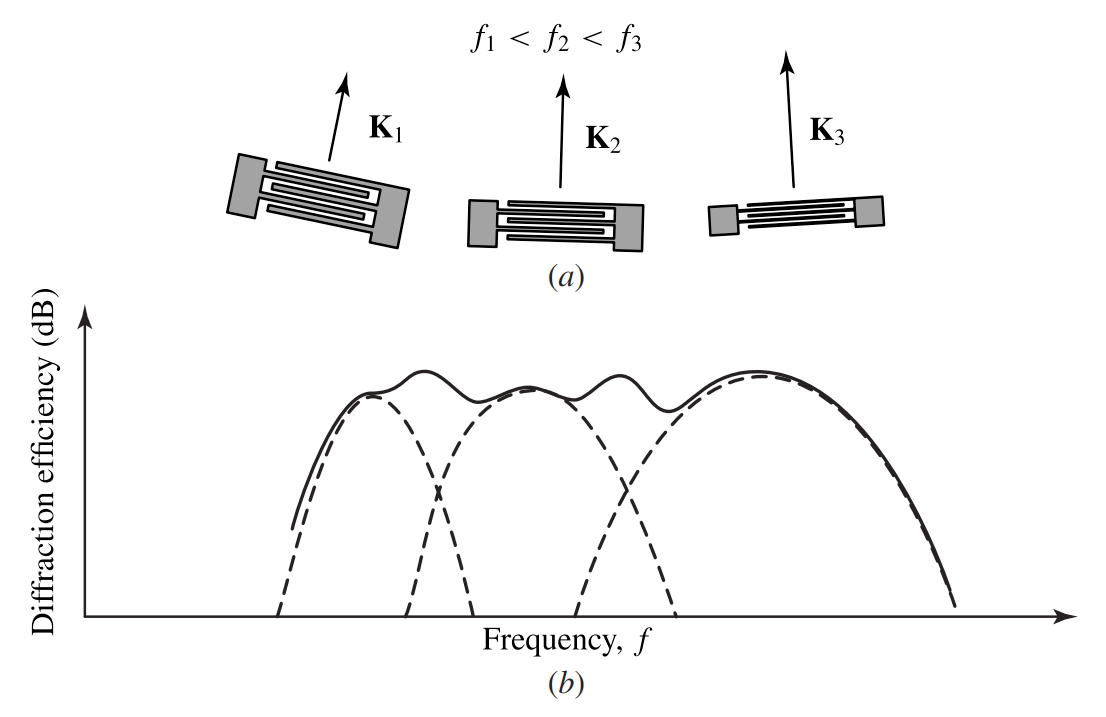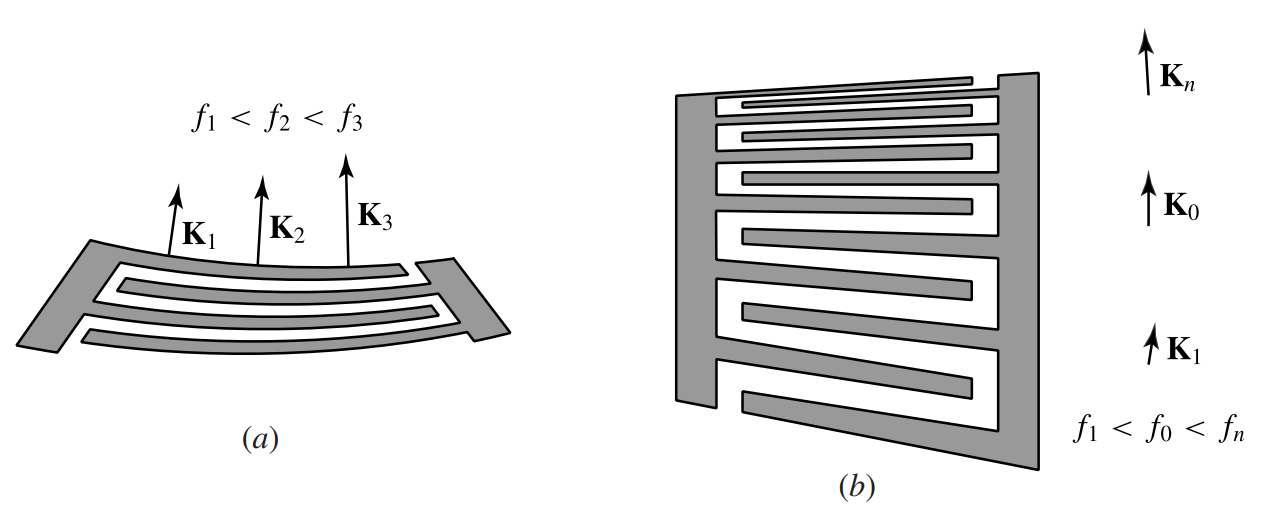# Guided-wave acousto-optic devices

This is a continuation from the previous tutorial - acousto-optic tunable filters.

Guided-wave acousto-optic devices are developed along the same principles discussed in the previous tutorials for bulk devices. For the same reasons as those that require practical bulk acousto-optic devices to be of Bragg type, all practical guided-wave acousto-optic devices also function in the Bragg regime. With only a few exceptions, a bulk counterpart can be identified for each guided-wave acousto-optic device, both of which have the same basic operation principles and are subject to the same considerations in terms of performance characteristics.

Guided-wave acousto-optic devices differ from bulk acousto-optic devices in two major aspects. First, the acoustic wave used in a guided-wave device is a surface acoustic wave (SAW) that is excited by a transducer fabricated on the top of the device rather than the volume acoustic wave that is used in a bulk device and is excited by a transducer attached to one end of the device. Furthermore, as is true for any guided-wave device, the optical waves involved in the function of a guided-wave acousto-optic device are in the form of waveguide modes.

A SAW needed in a guided-wave acousto-optic device is excited using an interdigital transducer (IDT) that consists of an array of electrodes on the piezoelectric surface of the device, as shown in figure 8-17 below.Figure 8-17. Generation of a surface acoustic wave by an interdigital transducer.

Many anisotropic crystals, such as LiNbO3, are piezoelectric. Both the IDT for generating the SAW and the optical waveguide can be fabricated directly on such a material. Isotropic materials, such as fused silica, are not piezoelectric. Many useful crystals, such as GaAs and quartz, are also non-piezoelectric. When a non-piezoelectric material is used as the waveguide substrate, a layer of piezoelectric material, such as ZnO, has to be placed on top for generation of a SAW through an IDT.

A SAW differs in many significant aspects from a bulk acoustic wave. These differences account for some qualitative and quantitative differences between a guided-wave acousto-optic device and its bulk counterpart. They also make the analysis of a guided-wave acousto-optic device complicated. Without getting into detailed analysis of SAWs, which in many cases of anisotropic media has no analytical solution, we consider here only some key features of SAWs. To facilitate the discussions, we consider a SAW propagating in the $$z$$ direction on the surface of a material normal to the $$y$$ direction, as shown in figure 8-17.

1. A SAW cannot be considered to be either longitudinal or transverse as a bulk acoustic wave is. Its mechanical displacement vector $$\mathbf{u}$$ has at least two components, and sometimes all three components, coupled together. Therefore, a SAW is always elliptically polarized. Moreover, the ellipticity of a SAW is a function of the distance $$y$$ into the substrate.

2. In the case of an isotropic medium and also in the case of an anisotropic medium with $$yz$$ being a crystal symmetry plane, $$\mathbf{u}$$ has only $$y$$ and $$z$$ components. We then have $$\mathbf{u}(\mathbf{r},t)=\hat{y}u_y(y,z,t)+\hat{z}u_z(y,z,t)=(\hat{y}U_y(y)+\hat{z}U_z(y))\cos(Kz-\Omega{t})$$ for a traveling SAW propagating in the $$z$$ direction, where both $$U_y(y)$$ and $$U_z(y)$$ decay in the $$y$$ direction in a distance on the order of the acoustic wavelength $$\Lambda$$. In this situation, we find that the nonvanishing strain tensor elements are $$S_2=S_{yy}$$, $$S_3=S_{zz}$$, and $$S_4=2S_{yz}$$.

3. In the case of an anisotropic medium with $$yz$$ not coinciding with any crystal symmetry plane, $$\mathbf{u}$$ has all three $$x$$, $$y$$, and $$z$$ components. All three components are functions of $$y$$ and $$z$$ but do not vary with $$x$$. Then, $$\mathbf{u}(\mathbf{r},t)=\hat{x}u_x(y,z,t)+\hat{y}u_y(y,z,t)+\hat{z}u_z(y,z,t)=(\hat{x}U_x(y)+\hat{y}U_y(y)+\hat{z}U_z(y))\cos(Kz-\Omega{t})$$. We find in this situation that only $$S_1=S_{xx}$$ vanishes. All other strain tensor elements exist at the same time.

4. While any strain tensor element $$S(y,z,t)$$ is a function of $$y$$, $$z$$, and $$t$$, its amplitude $$\mathcal{S}(y)$$ is a function of $$y$$ only because the amplitude $$U(y)$$ of the displacement is a function of $$y$$ only. The strain field of a SAW is localized in a surface layer of a thickness on the order of the acoustic wavelength, but it might oscillate as it tapers off. The amplitudes of different strain tensor elements normally have different magnitudes and phases as a SAW is elliptically polarized.

5. The total acoustic power of a SAW is then

$\tag{8-155}P_\text{a}=\frac{L}{2}\rho{v_\text{a}}^3\displaystyle\int\limits_0^\infty\mathcal{S}^2(y)\text{d}y$

where $$L$$ is the width of the SAW beam and $$v_\text{a}$$ is the velocity of the SAW. In a uniform IDT like the one shown in figure 8-17, $$L$$ is defined by the overlap between adjacent electrodes rather than by the length of the electrodes.

6. In a piezoelectric medium such as LiNbO3, acousto-optic interaction between a SAW and an optical wave generally involves the electro-optic effect caused by the electric field generated by the SAW. This phenomenon can have a significant effect on the figure of merit of the acousto-optic interaction.

7. The velocity of a SAW is smaller than the velocities of the longitudinal and transverse modes of the bulk acoustic wave in the same medium.

The polarity of the bias voltage alternates periodically in an IDT, resulting in a spatial periodicity that is characterized by a pair of electrodes of opposite polarity in each period. For an IDT that is designed to function around a center acoustic frequency of $$f_0$$, this periodicity has to match the wavelength $$\Lambda_0$$ of the SAW at this frequency. Thus the center-to-center distance between two adjacent electrodes of opposite polarity is $$d_\text{e}=\Lambda_0/2$$. The corresponding SAW frequency

$\tag{8-156}f_0=\frac{v_\text{a}}{2d_\text{e}}$

is called the synchronous frequency of the IDT.

To generate a SAW at a frequency $$f$$, which can be different from $$f_0$$, an RF signal at this frequency $$f$$ is applied to the electrodes of the IDT. As illustrated in figure 8-17, an IDT radiates bidirectionally two SAWs of equal power at both ends. Only one of these two SAWs is useful at a time for any given acousto-optic interaction in a device.

The conversion efficiency of an IDT is determined by the matching circuit that drives the IDT and the radiation impedance of the electrode array in the IDT. The frequency response of an IDT has a bandwidth that is determined by the number $$N_\text{e}$$ of the electrodes or, more precisely, the number $$N_\text{g}=N_\text{e}-1$$ of the gaps in the electrode array. Therefore, the useful acoustic power, which accounts for the acoustic output from only one end of the IDT, is

$\tag{8-157}P_\text{a}(f)=\eta_\text{t}(f,N_\text{g})P_\text{e}(f)=\eta_\text{ckt}(f,N_\text{g})\eta_\text{a}(f,N_\text{g})P_\text{e}(f)$

where $$P_\text{e}(f)$$ is the electrical power at the RF frequency $$f$$, $$\eta_\text{ckt}(f,N_\text{g})$$ accounts for the efficiency of the matching circuit, and

$\tag{8-158}\eta_\text{a}(f,N_\text{g})=\frac{\sin^2[N_\text{g}\pi(f-f_0)/2f_0]}{N_\text{g}^2\sin^2[\pi(f-f_0)/2f_0]}\approx\frac{\sin^2[N_\text{g}\pi(f-f_0)/2f_0]}{[N_\text{g}\pi(f-f_0)/2f_0)]^2}$

is the normalized acoustic radiation efficiency of the IDT.

This normalized efficiency has a unity peak value at the center frequency: $$\eta_\text{a}(f_0,N_\text{g})=1$$. The bandwidth of $$\eta_\text{a}$$ depends on the number of electrodes. From (8-158), we find that the 3-dB acoustic radiation bandwidth, $$f_\text{3dB}$$, of the IDT for $$\eta_\text{a}(f_0\pm{f_\text{3dB}}/2,N_\text{g})=1/2$$ is

$\tag{8-159}f_\text{3dB}=\frac{1.772}{N_\text{g}}f_0$

The specific functional form of $$\eta_\text{ckt}(f,N_\text{g})$$ depends on the type of circuit used to drive the IDT. Both its peak value and its bandwidth depend on the number of electrodes and the circuit design. For a given type of circuit, the peak efficiency increases but the bandwidth decreases as the number of electrodes in an IDT is increased. The circuit is usually designed to have a bandwidth much larger than the acoustic radiation bandwidth so that $$\eta_\text{ckt}$$ is practically a constant at its peak value in the frequency range within $$f_0\pm{f}_\text{3dB}/2$$. The theoretical maximum value of $$\eta_\text{ckt}$$ is $$1/2$$ because an IDT radiates bidirectionally. Therefore, in the most ideal situation with an optimized broadband circuit of maximum efficiency, we have $$\eta_\text{t}(f,N_\text{g})\le\eta_\text{a}(f,N_\text{g})/2$$ with a bandwidth limited by $$f_\text{3dB}$$ given in (8-159).

Example 8-10

A $$z$$-propagating SAW in $$y$$-cut LiNbO3 has a phase velocity of $$v_\text{a}=3.488\text{ km s}^{-1}$$. An IDT is designed to generate this SAW at a center frequency of 500 MHz and a 3-dB bandwidth of at least 100 MHz. Find the center-to-center separation between the electrodes and the number of electrodes for the IDT.

The synchronous frequency of the IDT has to be the center acoustic frequency $$f_0=500\text{ MHz}$$. Therefore, we find from (8-156) that the center-to-center electrode separation needs to be

$d_\text{e}=\frac{v_\text{a}}{2f_0}=\frac{3.488\times10^3}{2\times500\times10^6}\text{ m}=3.488\text{ μm}$

For $$f_\text{3dB}\ge100\text{ MHz}$$, we find from (8-159) that

$N_\text{g}=1.772\frac{f_0}{f_\text{3dB}}\le\frac{1.772\times500\times10^6}{100\times10^6}=8.86$

Therefore, the maximum value for $$N_\text{g}$$ is 8, and the maximum number of electrodes is $$N_\text{e}=9$$.

A guided-wave acousto-optic device can be either coplanar or collinear. Similarly to their bulk counterparts, guided-wave acousto-optic modulators and deflectors generally use small-angle Bragg diffraction in a coplanar configuration while guided-wave acousto-optic tunable filters and mode converters have the configuration of collinear Bragg diffraction.

The basic configurations for coplanar and collinear guided-wave acousto-optic devices are shown in figure 8-18(a) and (b), respectively.Figure 8-18. Basic configurations for (a) coplanar and (b) collinear guided-wave acousto-optic devices.

In either case, the phase-matching condition is dictated by the wavevectors of the participating normal modes of the optical waveguide:

$\tag{8-160}\beta_\text{d}=\beta_\text{i}\pm\mathbf{K}$

where $$\beta_\text{i}$$ and $$\beta_\text{d}$$ are the normal mode wavevectors of the incident and diffracted optical beams, respectively, $$\mathbf{K}$$ is the wavevector of the SAW, and the plus and minus signs are for up- and down-shifted diffraction, respectively. Most of the results obtained in the acousto-optic diffraction tutorial for Bragg diffraction in a bulk medium can be applied directly to Bragg diffraction in an optical waveguide by (1) replacing $$\mathbf{k}_\text{i}$$ and $$\mathbf{k}_\text{d}$$ by $$\beta_\text{i}$$ and $$\beta_\text{d}$$, respectively, and (2) interpreting $$n_\text{i}$$ and $$n_\text{d}$$ as $$n_\text{i}=\lambda\beta_\text{i}/2\pi$$ and $$n_\text{d}=\lambda\beta_\text{d}/2\pi$$ to represent the effective refractive indices seen by relevant waveguide modes. The phase mismatch, if it exists, becomes $$\Delta\mathbf{k}=\beta_\text{d}-\beta_\text{i}-\mathbf{K}$$ for up-shifted diffraction and $$\Delta\mathbf{k}=\beta_\text{d}-\beta_\text{i}+\mathbf{K}$$ for down-shifted diffraction. After making these modifications, the results obtained in the preceding tutorials for bulk acousto-optic devices are also valid for their guided-wave counterparts, with the exception of the diffraction efficiency and some other characteristics discussed later in this tutorial.

The coupled equations for Bragg diffraction in an optical waveguide have to be obtained by employing a coupled-mode analysis. They have the same form as (8-79) and (8-80) [refer to the acousto-optic diffraction tutorial], with $$A_\text{i}$$ and $$A_\text{d}$$ now representing the amplitudes of waveguide modes that characterize the incident and the diffracted optical beams, respectively. The Bragg coupling coefficient obtained from the coupled-mode analysis can be expressed in a form similar to that in (8-81) [refer to the acousto-optic diffraction tutorial]:

$\tag{8-161}\kappa=\pm\text{i}\frac{\omega^2\mu_0}{4\beta_\text{i}^{1/2}\beta_\text{d}^{1/2}}\Delta\tilde{\epsilon}_\text{id}^\text{eff}$

where the plus and minus signs are for up- and down-shifted diffraction, respectively, and

$\tag{8-162}\Delta\tilde{\epsilon}_\text{id}^{\text{eff}}=\frac{2\beta_\text{i}^{1/2}\beta_\text{d}^{1/2}}{\omega\mu_0}\displaystyle\int\limits_{-\infty}^{\infty}\hat{\pmb{\mathcal{E}}}_\text{i}^*\cdot\Delta\tilde{\pmb{\epsilon}}\cdot\hat{\pmb{\mathcal{E}}}_\text{d}\text{d}y$

If the photoelastic effect is the sole contribution to the changes in the dielectric properties of the medium in a guided-wave device, we have

$\tag{8-163}\Delta\tilde{\epsilon}_\text{id}^\text{eff}\approx-\epsilon_0\left(\frac{2n_\text{i}n_\text{d}M_2P_\text{a}}{L\Lambda}\right)^{1/2}\Gamma_\text{id}$

where $$n_\text{i}$$ and $$n_\text{d}$$ are the refractive indices seen by the modes of the incident and the diffracted optical beams, respectively, and

$\tag{8-164}\Gamma_\text{id}=\frac{\displaystyle\int\limits_0^\infty\mathcal{S}(y)\hat{\mathcal{E}}_\text{i}^*(y)\hat{\mathcal{E}}_\text{d}(y)\text{d}y}{\left[\frac{1}{\Lambda}\displaystyle\int\limits_0^\infty\mathcal{S}^2(y)\text{d}y\int\limits_{-\infty}^\infty|\hat{\mathcal{E}}_\text{i}(y)|^2\text{d}y\int\limits_{-\infty}^\infty|\hat{\mathcal{E}}_\text{d}(y)|^2\text{d}y\right]^{1/2}}$

is a dimensionless overlap factor for the optical modes and the strain field of the SAW. The overlap factor $$\Gamma_\text{id}$$ accounts for the different spatial distributions of the optical modes and the SAW. It is a function of the acoustic frequency $$f$$ because the strain field distribution of a SAW depends on the acoustic frequency. The Bragg coupling coefficient for a guided-wave acousto-optic device can then be expressed as

$\tag{8-165}|\kappa|=\frac{\pi}{\lambda}\left(\frac{M_2P_\text{a}}{2L\Lambda}\right)^{1/2}\Gamma_\text{id}=\frac{\pi}{\lambda}\left(\frac{M_2fP_\text{a}}{2Lv_\text{a}}\right)^{1/2}\Gamma_\text{id}$

Consequently, for a coplanar or a codirectional, collinear guided-wave acousto-optic device that uses a traveling acoustic wave, the diffraction efficiency for an interaction length $$l$$ in the case of perfect phase matching is

$\tag{8-166}\eta_\text{PM}=\sin^2\left[\frac{\pi}{\lambda}\left(\frac{M_2f}{2Lv_\text{a}}P_\text{a}(f)\right)^{1/2}\Gamma_\text{id}(f)l\right]$

or, in the low-efficiency limit,

$\tag{8-167}\eta_\text{PM}\approx\frac{\pi^2M_2l^2f}{2\lambda^2Lv_\text{a}}\Gamma_\text{id}^2(f)P_\text{a}(f),\qquad\text{if }\eta_\text{PM}\ll1$

In expressing $$\Delta\tilde{\epsilon}_\text{id}^\text{eff}$$ in the form of (8-163), we have assumed that only the photoelastic effect contributes to the changes in the dielectric properties of the medium. The discussions and conclusions that follow (8-163) are subject to this assumption.

Many practical acousto-optic materials, such as LiNbO3 for example, are piezoelectric and have large Pockels coefficients. Through the piezoelectric effect, the SAW can generate a significant electric field, which can then induce a significant change in the dielectric properties of the medium through the Pockels effect. In this situation, $$\Delta\tilde{\pmb{\epsilon}}$$ has contributions from both photoelastic and electro-optic effects. Then, the parameters $$M_2$$ and $$\Gamma_\text{id}$$ that appear in (8-165)-(8-167) have contributions from both effects. In certain situations, such as in the interaction of a TE mode with a $$z$$-propagating SAW in a $$y$$-cut LiNbO3 waveguide, the contribution from the electro-optic effect can even dominate that from the photoelastic effect.

Modulators

A guided-wave acousto-optic modulator generally has a coplanar configuration like that shown in figure 8-18(a) above. The general characteristics of acousto-optic modulators discussed in the acousto-optic modulators tutorial remain true for guided-wave modulators as well. The only characteristic that is different between a guided-wave acousto-optic modulator and its bulk counterpart is the functional dependence of the diffraction efficiency on various device parameters, as is discussed above and can be seen by comparing (8-166) with (8-106). Although a modulator is driven by a time-dependent signal at a fixed carrier frequency, the diffraction efficiency of each frequency component in the modulation signal varies because of its frequency dependence, which is expressed in (8-166). As a result, the modulation bandwidth of a guided-wave acousto-optic modulator is not only determined by the acoustic transit time $$\tau_\text{a}$$, but is also subject to the bandwidth of the IDT determined by (8-158) and that of the overlap factor $$\Gamma_\text{id}(f)$$.

Deflectors

Guided-wave acousto-optic deflectors are coplanar devices. They have a basic configuration like that shown in figure 8-18 (a) above though more sophisticated transducers are often used for broadband deflectors. The discussions in the acousto-optic deflectors tutorial are generally applicable to guided-wave acousto-optic deflectors.

The most important parameter that characterizes the performance of an acousto-optic deflector is its bandwidth $$\Delta f$$ because, as the relation in (8-132) [refer to the acousto-optic deflectors tutorial] shows, the number of spatially resolvable spots that can be addressed by a deflector is linearly proportional to its bandwidth. The bandwidth of a guided-wave acousto-optic deflector is limited by the phase-matching bandwidth of the acousto-optic Bragg diffraction, the IDT bandwidth, and the bandwidth of the overlap factor.

The characteristics of the Bragg phase-matching bandwidth have been fully discussed in the acousto-optic deflectors tutorial. The Bragg bandwidth can be increased either by using birefringent Bragg diffraction under the tangential phase-matching condition or by using a phased-array transducer in a nonbirefringent deflector. These approaches are applicable to guided-wave deflectors as well.

In a birefringent guided-wave Bragg deflector that uses the tangential phase-matching condition, the incident and the diffracted optical beams are waveguide modes of orthogonal polarizations. In a planar waveguide, this kind of interaction involves coupling between TE and TM modes. A bandwidth comparable to that of an optimized birefringent deflector is possible for a nonbirefringent deflector that uses a phased-array transducer.

In a guided-wave device, a phased-array transducer consists of multiple elements of identical IDTs that are equally spaced but are successively displaced at a constant step $$h$$, as shown in figure 8-19 below. The step height is chosen to be an integral multiple of the center-to-center distance between adjacent electrodes:

$\tag{8-168}h=md_\text{e}=m\frac{\Lambda_0}{2},\qquad m=\text{integer}$Figure 8-19. Phased-array interdigital transducer for the generation of a surface acoustic wave whose propagation direction is steered by the acoustic frequency to track the Bragg condition.

At the center frequency $$f_0$$, the SAWs generated by the IDT elements are all in phase, resulting in a combined SAW propagating in the normal direction. At any frequency $$f$$ shifted away from $$f_0$$, the regular spatial displacement between successive IDT elements causes a frequency-dependent regular phase shift between adjacent individual SAWs, thereby steering the direction of the combined SAW wavefront according to the variation in the acoustic frequency.

As can be seen from (8-158) and subsequent discussions, the bandwidth of a regular IDT is quite limited. A phased-array IDT as shown in figure 8-19 increases the Bragg bandwidth of the acousto-optic interaction, but it does not improve the bandwidth of the IDT itself. Consequently, the bandwidth of a deflector using such a phased-array IDT is still subject to the limitation of the same IDT bandwidth.

This limitation can be overcome by using an array of multiple tilted IDTs of staggered synchronous frequencies, as shown in figure 8-20(a).Figure 8-20. (a) Multiple tilted interdigital transducers of staggered center frequencies. (b) Individual frequency responses and composite frequency response of the transducers.

The individual IDTs are tilted in order for the Bragg phase-matching condition to be satisfied at each synchronous frequency. The difference in the tilt angles between two adjacent IDTs is equal to the difference between the Bragg angles at the corresponding synchronous frequencies of the two IDTs.

The frequency response of each individual IDT is centered at the corresponding synchronous frequency. The synchronous frequencies of the individual IDTs are staggered in such a way that the individual frequency responses of adjacent IDTs intersect at -6 dB down from the peak response in order to obtain a large composite overall frequency response, as illustrated in figure 8-20(b).

Two broadband IDT structures that evolve from the same concept are shown in figure 8-21 below.Figure 8-21. (a) Curved interdigital transducer with tapered electrodes. (b) Tilted-finger chirped interdigital transducer.

The curved IDT with tapered electrodes shown in figure 8-21(b) results from parallel connection of multiple tilted IDTs of continuously varying parameters, whereas the tilted-finger chirped IDT shown in figure 8-21(b) results from serial stacking of multiple tilted IDTs of continuously varying parameters.

An array of multiple tilted IDTs has a broad composite transducer bandwidth. It also provides a broad Bragg bandwidth due to tracking of the Bragg phase-matching condition by the tilted angles of the individual IDTs. The curved IDT and the tilted-finger chirped IDT have similar characteristics.

In contrast, a phased-array IDT increases only the Bragg bandwidth but not the transducer bandwidth. However, at any given acoustic frequency, all of the elements in a phased-array IDT are simultaneously excited, whereas in any of the broadband IDTs only part of the structure is effectively excited at a given acoustic frequency due to the design of staggered synchronous frequencies. Therefore, a deflector that uses a phased-array IDT can have a larger diffraction efficiency than one that uses one of the broadband IDTs.

In order to optimize the bandwidth and the efficiency at the same time, a combination of different schemes is often used. For example, an array of multiple tilted phased-array IDTs of staggered synchronous frequencies can be designed for a nonbirefringent deflector of high efficiency and large bandwidth. In a birefringent deflector that has a large Bragg bandwidth, the Bragg angle varies little with varying acoustic frequencies due to the tangential phase-matching scheme. For such a device to have a large overall bandwidth, its Bragg bandwidth can be matched with a large IDT bandwidth by using an array of multiple untilted IDTs of staggered synchronous frequencies or a parallel-finger chirped IDT.

To utilize the Bragg bandwidth and the IDT bandwidth fully, the bandwidth of the overlap factor is generally made large enough by ensuring, through the design of the waveguide structure, sufficient overlap of the interacting optical modes with the SAW field distribution.

Tunable Filters

Guided-wave acousto-optic tunable filters are typically collinear devices though, as is mentioned in the acousto-optic tunable filters tutorial, noncollinear configurations are also possible.

The general discussions presented in the acousto-optic tunable filters tutorial apply to guided-wave tunable filters as well. However, guided-wave tunable filters have some unique characteristics due to modal dispersion that does not exist in bulk devices.

Because modes of the same polarization but different orders, such as TE0 and TE1, have different propagation constants, a collinear guided-wave acousto-optic tunable filter that is based on a change in the mode order without a change in the polarization is possible.

However, guided-wave acousto-optic tunable filters that involve orthogonally polarized modes cannot be constructed with isotropic materials though the orthogonally polarized TE and TM modes always have different propagation constants even when the waveguide structure is made of layers of isotropic materials.

It is possible to construct collinear acousto-optic filters with TE-TM mode conversion using waveguides in properly oriented anisotropic substrates.

Mode Converters

The guided-wave acousto-optic filters discussed above can be used as guided-wave acousto-optic mode converters. The only difference between these two types of devices is the purpose of their applications.

Any guided-wave acousto-optic tunable filter cannot operate without converting one waveguide mode to another, but is purpose is to select a desired optical wavelength out of a possibly broad spectrum.

In contrast, the functional purpose of a guided-wave acousto-optic mode converter is to convert a waveguide mode from one polarization to another or from one mode order to another.

A high mode-conversion efficiency is the most desirable characteristic of a mode converter. A mode converter is generally designed for the phase-matching condition to be satisfied at a desired optical wavelength of application. Furthermore, the input to the device is a coherent optical wave in the designated incident waveguide mode at this particular wavelength so that a high mode-conversion efficiency can be obtained.

The next part continues with the optical nonlinearity tutorial